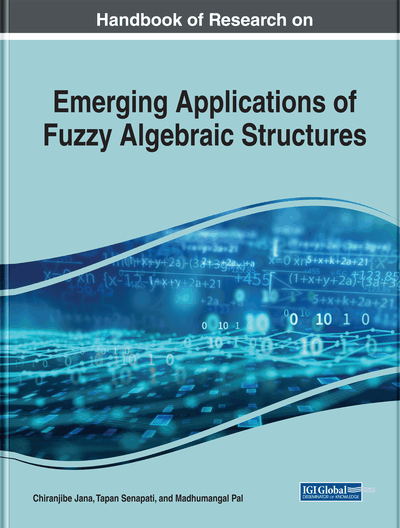Fuzzy Translation of Doubt Interval-Valued Fuzzy Ideals in BF-Algebras

Tripti Bej (Vidyasagar University, India) and Young Bae Jun (Gyeongsang National University, South Korea)
DOI: 10.4018/978-1-7998-0190-0.ch014
Available
\$37.50
No Current Special Offers

Abstract

This chapter focuses on the basics of a theory for such an interval-valued fuzzy set becoming doubt interval-valued fuzzy subalgebra and doubt interval-valued fuzzy ideal in BF-algebras. After a detailed study of its properties, the authors come to the conclusion that in BF-algebras an interval-valued fuzzy set in BF-algebras is a doubt interval-valued fuzzy subalgebra and a doubt interval-valued fuzzy ideal if and only if the complement of this interval-valued fuzzy set is an interval-valued fuzzy subalgebra and an interval-valued fuzzy ideal.
Chapter Preview
Top

1. Introduction

It is known that mathematical logic is a discipline used in sciences and humanities with different point of views. Non-classical logic takes the advantage of the classical logic (two-valued logic) to handle information with various facts of uncertainty. The non-classical logic has become a formal and useful tool for computer science to deal with fuzzy information and uncertain information. The notion of logical algebras: BCK algebras and BCI algebras were initiated by Imai and Iseki (1966) in 1966 as a generalization of both classical and non-classical positional calculus. It is known that the class of BCK algebras is a proper subclass of the class of BCI algebras. In 2007, Andrzej Walendziak defined the BF algebra (Walendziak, A., 2007).

The work of Zadeh (1975) made an extension of the concept of a fuzzy set by an interval-valued fuzzy set (i.e., a fuzzy set with an interval-valued membership function). An interval-valued fuzzy set is a fuzzy set whose membership function is many-valued and forms an interval with respect to the membership scale. Biswas (1994) defined interval-valued fuzzy sub groups.

To develope the theory of BCK/BCI algebras the ideal theory plays an important role. Jun, Meng, Liu and several researchers investigated properties of fuzzy subalgebras and ideals in BCK/BCI algebras (Jun, Y. B., 1993; Jun, Y. B., 1994; Meng, J., 1994; Liu, Y, L. and Meng, J. 2001; Meng, J. and Guo, X., 2005; Bej, T. and Pal, M., 2014a; Bej, T. and Pal, M. 2014b; Bej, T. and Pal, M. 2015). Zarandi and Saeid (Zarandi, A. and Saeid, A. B., 2009) applied the notion of interval-valued fuzzy to BF subalgebras.

Jun (1994) introduced the notion of doubt fuzzy subalgebras and doubt fuzzy ideals in BCK/BCI algebras. Biswas (1990) introduced the concept of anti fuzzy subgroup. Modifying their idea, Saeid (2011) introduced the notion of doubt fuzzy subalgebra of BF algebras and Barbhuiya (2014) introduced the notion of doubt fuzzy ideal of BF algebras.

The authors have studied doubt intuitionistic fuzzy ideals in BCK/BCI algebras, doubt intuitionistic fuzzy H-ideals in BCK/BCI algebras and doubt Atanassov's intuitionistic fuzzy subimplicative-ideals in BCI algebras in their earlier works (Bej, T. and Pal, M. 2014a; Bej, T. and Pal, M. 2014b; Bej, T. and Pal, M. 2015).

Following the discussion of Barbhuiya (2014); Senapati, T., Bhowmik, M. & Pal, M. (2012c); Senapati, T., Bhowmik, M. & Pal, M.. (2013) authors are going to introduce the concept of doubt interval-valued fuzzy ideals in BF algebras. After a detailed study of its properties, they come to this conclusion that in BF algebras, an interval-valued fuzzy set is a doubt interval-valued fuzzy ideal in BF algebras if and only if the complement of this interval-valued fuzzy set is an interval-valued fuzzy ideal. Finally, results based on doubt interval-valued fuzzy subalgebras of BF algebras and doubt interval-valued fuzzy ideals of BF algebras are established. At the same time the product of doubt interval-valued fuzzy ideals in BF algebras has been introduced. Besides the idea of fuzzy translation, fuzzy multiplication of a doubt interval-valued fuzzy subalgebra and doubt interval-valued fuzzy ideal of a BF-algebra are given along with several related characterisation theorems.

Top

2. Preliminaries

In this section, some elementary aspects that are necessary for this paper are included.

Complete Chapter List

Search this Book:
Reset